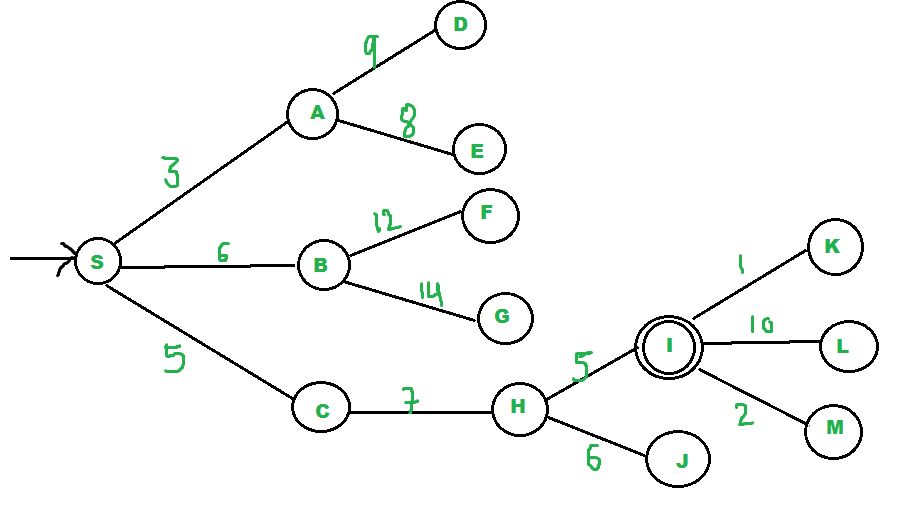# Best First Search (Informed Search)

• Difficulty Level : Easy
• Last Updated : 12 Oct, 2021

Prerequisites : BFS, DFS
In BFS and DFS, when we are at a node, we can consider any of the adjacent as next node. So both BFS and DFS blindly explore paths without considering any cost function. The idea of Best First Search is to use an evaluation function to decide which adjacent is most promising and then explore. Best First Search falls under the category of Heuristic Search or Informed Search.

We use a priority queue to store costs of nodes. So the implementation is a variation of BFS, we just need to change Queue to PriorityQueue.

Attention reader! Don’t stop learning now. Get hold of all the important DSA concepts with the DSA Self Paced Course at a student-friendly price and become industry ready.  To complete your preparation from learning a language to DS Algo and many more,  please refer Complete Interview Preparation Course.

In case you wish to attend live classes with experts, please refer DSA Live Classes for Working Professionals and Competitive Programming Live for Students.

```// This pseudocode is adapted from below
// source:
// https://courses.cs.washington.edu/
Best-First-Search(Graph g, Node start)
1) Create an empty PriorityQueue
PriorityQueue pq;
2) Insert "start" in pq.
pq.insert(start)
3) Until PriorityQueue is empty
u = PriorityQueue.DeleteMin
If u is the goal
Exit
Else
Foreach neighbor v of u
If v "Unvisited"
Mark v "Visited"
pq.insert(v)
Mark u "Examined"
End procedure```

Let us consider the below example.```We start from source "S" and search for
goal "I" using given costs and Best
First search.

pq initially contains S
We remove s from and process unvisited
neighbors of S to pq.
pq now contains {A, C, B} (C is put
before B because C has lesser cost)

We remove A from pq and process unvisited
neighbors of A to pq.
pq now contains {C, B, E, D}

We remove C from pq and process unvisited
neighbors of C to pq.
pq now contains {B, H, E, D}

We remove B from pq and process unvisited
neighbors of B to pq.
pq now contains {H, E, D, F, G}

We remove H from pq.  Since our goal
"I" is a neighbor of H, we return.```

Below is the implementation of the above idea:

## C++

 `// C++ program to implement Best First Search using priority``// queue``#include ``using` `namespace` `std;``typedef` `pair<``int``, ``int``> pi;` `vector > graph;` `// Function for adding edges to graph``void` `addedge(``int` `x, ``int` `y, ``int` `cost)``{``    ``graph[x].push_back(make_pair(cost, y));``    ``graph[y].push_back(make_pair(cost, x));``}` `// Function For Implementing Best First Search``// Gives output path having lowest cost``void` `best_first_search(``int` `source, ``int` `target, ``int` `n)``{``    ``vector<``bool``> visited(n, ``false``);``    ``// MIN HEAP priority queue``    ``priority_queue, greater > pq;``    ``// sorting in pq gets done by first value of pair``    ``pq.push(make_pair(0, source));``    ``int` `s = source;``    ``visited[s] = ``true``;``    ``while` `(!pq.empty()) {``        ``int` `x = pq.top().second;``        ``// Displaying the path having lowest cost``        ``cout << x << ``" "``;``        ``pq.pop();``        ``if` `(x == target)``            ``break``;` `        ``for` `(``int` `i = 0; i < graph[x].size(); i++) {``            ``if` `(!visited[graph[x][i].second]) {``                ``visited[graph[x][i].second] = ``true``;``                ``pq.push(make_pair(graph[x][i].first,graph[x][i].second));``            ``}``        ``}``    ``}``}` `// Driver code to test above methods``int` `main()``{``    ``// No. of Nodes``    ``int` `v = 14;``    ``graph.resize(v);` `    ``// The nodes shown in above example(by alphabets) are``    ``// implemented using integers addedge(x,y,cost);``    ``addedge(0, 1, 3);``    ``addedge(0, 2, 6);``    ``addedge(0, 3, 5);``    ``addedge(1, 4, 9);``    ``addedge(1, 5, 8);``    ``addedge(2, 6, 12);``    ``addedge(2, 7, 14);``    ``addedge(3, 8, 7);``    ``addedge(8, 9, 5);``    ``addedge(8, 10, 6);``    ``addedge(9, 11, 1);``    ``addedge(9, 12, 10);``    ``addedge(9, 13, 2);` `    ``int` `source = 0;``    ``int` `target = 9;` `    ``// Function call``    ``best_first_search(source, target, v);` `    ``return` `0;``}`

## Python

 `from` `queue ``import` `PriorityQueue``v ``=` `14``graph ``=` `[[] ``for` `i ``in` `range``(v)]` `# Function For Implementing Best First Search``# Gives output path having lowest cost`  `def` `best_first_search(source, target, n):``    ``visited ``=` `[``0``] ``*` `n``    ``visited ``=` `True``    ``pq ``=` `PriorityQueue()``    ``pq.put((``0``, source))``    ``while` `pq.empty() ``=``=` `False``:``        ``u ``=` `pq.get()[``1``]``        ``# Displaying the path having lowest cost``        ``print``(u, end``=``" "``)``        ``if` `u ``=``=` `target:``            ``break` `        ``for` `v, c ``in` `graph[u]:``            ``if` `visited[v] ``=``=` `False``:``                ``visited[v] ``=` `True``                ``pq.put((c, v))``    ``print``()` `# Function for adding edges to graph`  `def` `addedge(x, y, cost):``    ``graph[x].append((y, cost))``    ``graph[y].append((x, cost))`  `# The nodes shown in above example(by alphabets) are``# implemented using integers addedge(x,y,cost);``addedge(``0``, ``1``, ``3``)``addedge(``0``, ``2``, ``6``)``addedge(``0``, ``3``, ``5``)``addedge(``1``, ``4``, ``9``)``addedge(``1``, ``5``, ``8``)``addedge(``2``, ``6``, ``12``)``addedge(``2``, ``7``, ``14``)``addedge(``3``, ``8``, ``7``)``addedge(``8``, ``9``, ``5``)``addedge(``8``, ``10``, ``6``)``addedge(``9``, ``11``, ``1``)``addedge(``9``, ``12``, ``10``)``addedge(``9``, ``13``, ``2``)` `source ``=` `0``target ``=` `9``best_first_search(source, target, v)` `# This code is contributed by Jyotheeswar Ganne`
Output
`0 1 3 2 8 9 `

Analysis :

• worst case time complexity for Best First Search is O(n * Log n) where n is number of nodes. In worst case, we may have to visit all nodes before we reach goal. Note that priority queue is implemented using Min(or Max) Heap, and insert and remove operations take O(log n) time.
• Performance of the algorithm depends on how well the cost or evaluation function is designed.

Related Article:
A* Search Algorithm
This article is contributed by Shambhavi Singh. If you like GeeksforGeeks and would like to contribute, you can also write an article using write.geeksforgeeks.org or mail your article to review-team@geeksforgeeks.org. See your article appearing on the GeeksforGeeks main page and help other Geeks.